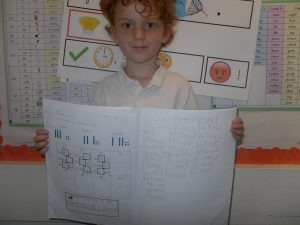# Perfect Partioning

In maths we have been partitioning numbers into tens and ones.

When we have the number 41, we know that it is 4 tens and 1 one.    We can write it 41 = 40 + 1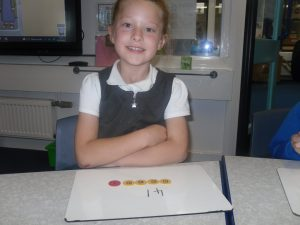Then we looked at partitioning numbers in different ways.

52 = 50 + 3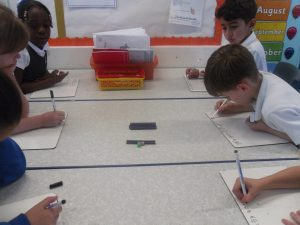If we move across a ten to the ones we have made

53 = 40 + 13

We moved 2 tens across and made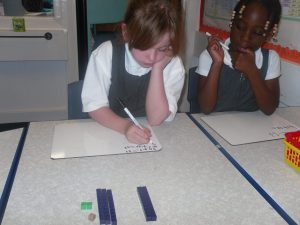53 = 20 + 33

Great job!

We also used tens and ones counters to help us work out the missing numbers.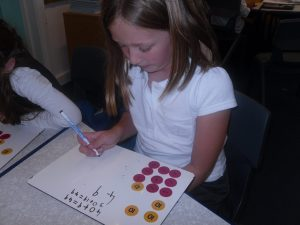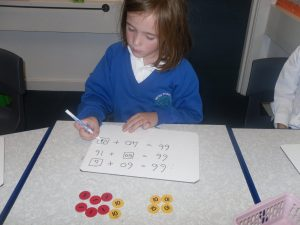We then applied our practical work to solve problems in our books.